Note: This article has never been published. It originally appeared as Fels Discussion Paper #52, University of Pennsylvania, March 1974. What is reproduced here is a later revision; I do not know its exact date. I have corrected a few typographical errors in the equations, but otherwise left the article unchanged. Anyone interested in publishing it, or continuing the line of research, should get in touch with me.

### ABSTRACT

I assume players of bilateral monopoly, to demonstrate their resolution, maintain a consistent strategy over many plays with different opponents. A semi-solution is a distribution of players over strategies such that no player can profit by changing his strategy; a solution is a semi-solution such that the players cannot all profit by altering their strategies slightly to an "adjacent" semi-solution. I explore the semi-solutions following from one definition of "consistent strategy" and find a unique solution; other definitions would produce different results.

### BILATERAL MONOPOLY: A SOLUTION*

David Friedman

Virginia Polytechnic Institute and State University

*The assistance of Professor Gerald Porter of the Mathematics Department of the University of Pennsylvania is gratefully acknowledged.

#### INTRODUCTION

Bilateral monopoly is a simple two person variable sum game. Its solution, in the classical game theory of Von Neumann and Morgenstern, contains an uncountable number of terms, representing all possible divisions of the profits from the game between the two players. The bargaining theory of Nash gives a unique solution, but it does so only by assuming that a symmetrical game must have a unique, symmetrical, and efficient solution, which in the case of bilateral monopoly amounts to assuming the answer. A number of other authors have derived the same unique solution from different sets of assumptions. This essay restates the problem in a form which preserves its essential characteristics, adds an additional feature which I believe to be an essential part of the real situation the game is intended to describe, and yields a unique solution.

#### PRELIMINARY CONSIDERATIONS

Bilateral monopoly is a two person game whose sum (which I call the surplus) is positive if the players reach an agreement on its division, otherwise zero. It is always in the immediate interest of either player to reach some agreement rather than none, provided that the share of the surplus allocated to him is greater than zero--the amount he gets if there is no agreement. So the division of the surplus appears indeterminate.

If we consider only a single play of the game, this argument may be sound. But a player who expects to be a participant in many such may reason that to accept a low share of the surplus in one game (the alternative being no agreement) will mark him as a "weak bargainer." His partners in future plays will then insist on getting the bulk of the surplus. By refusing to accept an unfavorable offer in the first play, he convinces future partners that if they do not offer him a reasonable share of the surplus there will be no agreement and neither partner will get anything. This may more than compensate him for the initial loss. To say the same thing in a different way, the player may find that even though the games are technically independent a single strategy for all of them is more profitable than separate strategies for each. Such a conclusion is paradoxical within the structure of formal game theory, yet intuitively plausible.

What I am here introducing into the theory is the idea of consistent behavior. I believe that players perceive certain patterns of behavior as consistent and that a player, by following a consistent strategy over many plays of the game, significantly strengthens his position. His resolution on each play is validated by the previous plays and by the necessity to continue to behave consistently in order that future plays will continue to be so validated. This element of consistency is precisely what is reflected in the idea of a weak or strong bargainer. A weak bargainer is one who consistently accepts a bad deal, a strong bargainer is one who consistently holds out for a good deal.

What behavior is considered consistent is not part of the rules of the game, nor is it a fact about the physical world external to the players. It is rather a fact about how the players organize their perception of behavior. One cannot, without knowing something about how the players think, "find out" what is or is not consistent behavior. In this essay I assume a particular perception of consistency, and from it derive a unique solution to the game. As I shall show later, the same theory, combined with a different perception of consistent behavior, would yield an entirely different solution.

Some readers may be disturbed by the introduction of a subjective element (beyond the subjective element already present in the idea of utility) into game theory. I suggest that such readers consider the "tacit" games described by Schelling, games whose solution depends on the patterns by which the players organize the data of the game. I suggest that they also consider whether what I call consistency is not an essential element of the real bargaining situation.

In actual bargaining each player may make a series of offers, each time trying to persuade his partner that his current offer is his last. In building the model I abstract away from such haggling by assuming that what really determines the outcomes ("on average") is the actual level, for each bargainer, below which he would prefer no agreement to accepting the other's offer, and that this level is an element (in this specific model the only element) of the player's strategy.

#### THE MODEL

In order to avoid confusion in terminology I will consider "bilateral monopoly" to be the name of the game and refer to the playing of the game as a "play." When I speak of a player playing bilateral monopoly several times with different opponents I am not, as an economist might suppose, describing an oligopoly, in which if the player cannot get what he wants from one person he can get it from another. The economist should rather imagine that in each play one partner has a unique item and the other player is the only person who wants that item. In each play the item is different.

I consider a group of persons each of whom will over time have many plays of the game, his partners being a random selection from the group. For simplicity, I assume that the surplus of the game is one dollar for each play--although of course that surplus will not be collected, for any particular play, unless the players reach agreement.

I limit the players to the following strategy. Each must select some part of the surplus upon which he will always insist. If, on a given play, the demands of the two partners are inconsistent (i.e., they total more than the one dollar surplus) there is no agreement, and nobody gets anything. If they are consistent, each player receives his demand and the remaining surplus is divided equally between them.

Each player knows the statistical distribution of the other players' strategies and may at any time use that knowledge to alter his own strategy in order to increase his profit. Players may not, however, alter their strategy according to what they know of the strategy of the partner they will next play. For if a player could do so, his partner could alter his strategy just before the play, demanding, say, 95% of the surplus, on the theory that the first player would lower his demand to 5%, preferring that to nothing. But then the first player would first raise his strategy to demanding 95%. Then the second ... this leads back to the sort of haggling which was assumed away earlier on the theory that what determines the outcome is what the player is actually willing to accept (on the basis of a profit maximizing long term strategy) rather than the persuasiveness of his bargaining offers.

An alternative way of justifying this assumption is to argue that a player who alters his demands according to his opponent is vulnerable to a sort of bargaining pressure that cannot be used against one who does not. The latter can "prove" his resolution by pointing out that yielding on one play vitiates his entire long term strategy. The former cannot.

This assumes that the players perceive "demanding a certain fraction of the surplus" as consistent behavior. A player who gradually modifies his demand in response to the general distribution of other players' demands is also consistent, or at least becomes consistent after he has stuck to his new strategy for a reasonable length of time. Since the game is played many times, short run losses while he is adjusting his strategy may be ignored.

I define a solution as a distribution of strategies which meets certain stability requirements. In stating these requirements I assume that the number of players is large, so that some distributions can be represented by continuous functions. (Obviously there are some distributions, such as everyone choosing the same strategy, which can never be so represented, however many players there are.)

I first define a semi-solution as a distribution of strategies such that:

1. Any strategy which is being played must yield the same expected return as any other such strategy.

The justification for this is that otherwise those playing the less attractive strategies would switch to the more attractive.

2. Any strategy which nobody is playing must be no more attractive than the strategies which are being played.

The justification is that otherwise players would shift to the unplayed strategies.

These semi-solutions are the Nash equilibria of the many player game --the sets of strategies such that it does not pay any player to change his strategy as long as all the others stick to theirs. As I show in the appendix, semi-solutions not only are not unique, they are very numerous. If they are my final solution concept I have made no improvement on the infinite solutions of Von Neumann and Morgenstern.

They are not. The semi-solutions are equilibria but, with a single exception, they are unstable equilibria. They should be thought of not as final distributions of strategies with which the players will be content forever but rather as distributions through which the players pass on their "search" for the final, stable solution.

A distribution which is not a semi-solution is unstable in a strong sense. There are players who can immediately profit by changing their strategy. It is reasonable to expect that the distribution of strategies will deviate from being a semi-solution for only very short periods of time.

A semi-solution is unstable in a more subtle way. No single player can profit by changing his strategy. But if many players change their strategies very slightly, all will be better off. It is in this sense that we speak of an unstable equilibrium.

Consider a pencil balanced on its point. It is in equilibrium; net forces on it are zero and (equivalently) no infinitesimal displacement can lower its potential energy. But disturb it the tiniest bit and it falls over.

A closer analogy is shown by figure 1. A ball is resting on the topmost of a series of rounded steps. The points A, B, and C are level, hence a ball resting at them is in (unstable) equilibrium. The rough surfaces a, b, and c, provide just enough friction so that a ball rolling from A will stop at B, from B at C, etc. Other surfaces are frictionless. The ball is in equilibrium, but, given the tiniest amount of vibration, will eventually find itself at the bottom of the steps.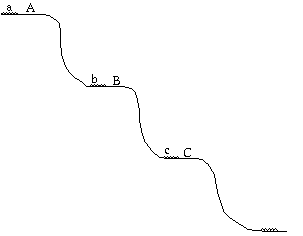Figure 1

This analogy is imperfect, since our problem works with many more dimensions; the semi-solutions which correspond to points A, B, C, are infinite in number and infinitely close together. But the logic of the system is the same, save that we are maximizing return instead of minimizing potential energy. Equilibrium points which can be "improved" by an infinitesimal disturbance are ultimately unstable.

I define a solution as a semi-solution such that:

3. There is no way of continuously altering the distribution such that the expected return to every player increases continuously during the alteration, and such that, during the alteration, the distribution is always a semi-solution.

As is shown in the appendix, the only solution is a distribution in which every player demands half of the surplus. All other initial distributions can and will be continuously deformed into it.

#### A DIFFERENT CONSISTENCY ASSUMPTION

So far I have assumed that players perceive as consistent a strategy of always demanding the same fraction of the surplus. Suppose instead that all players perceive utility as "real" and money merely as an uninteresting intermediary. Further suppose that they all believe that the marginal utility of money is inversely proportional to income. A policy of always demanding a fixed fraction of the money will seem to them very inconsistent, for a fixed fraction of the money is a larger or smaller fraction of the total utility produced by the game according to whether one player is poorer or richer than the other (assume incomes vary from player to player and are large compared to the profits from the game). Consistent behavior consists of always demanding the same fraction of the utility produced by the game. An even split of the money not only is not the solution for such a group of players, it is not even in their set of consistent strategies. Their unique solution is instead an even division of utility - which is to say, a division of the profits proportional to the income of the players. If we expand the theory to include the possibility of changing people's perceptions as a means of changing the solution, we conclude that it may pay rich people to subsidize the teaching of utility theory.

#### APPLICATION

Some readers may wonder what, if anything, the analysis applies to. I believe that it describes bilateral monopoly transactions as they actually occur in both economic and social interactions. The difficulty in under- standing such transactions comes from the attempt to understand them singly. But a businessman who in some particular situation finds himself part of a temporary bilateral monopoly, the only seller or the only buyer of a unique good (where the uniqueness may well be a matter of time and place, and not of intrinsic qualities), expects to find himself in similar situations many times again. In a community where the others with whom he will deal are likely to be aware of his previous dealings, his strategy is affected by his desire to maintain his bargaining reputation. Similarly an individual, in his social dealings, knows that to give in too easily on one issue makes him vulnerable to another, while a policy of always demanding the most he can hope for may lead to a solitary life, devoid of trading partners. It is to describe such situations that the model is designed.

#### APPENDIX: THE MATHEMATICS

I first consider the simple case where every player chooses one of three strategies, demanding either .4, .5, or .6 of the surplus.

Let N.4 =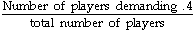Similarly N.5, N.6

Clearly N.4 +N.5+N.6=1

Let the expected return from one play of a player who demands .4 be

P.4 =.5 x N.4 + .45 x N.5 + .4 x N.6

Here .5 is the payoff if the opponent also demands .4 and N.4 the probability of meeting such an opponent. The other terms are similar. Then

P.5 =.55 x N.4 + .50 x N.5

P.6= .6 x N.4

By condition I

P.4 = P.5 =P.6= C provided that

.4 .5 .6 N.4, N.5, N.6 != 0.

By the definition of PN, C > 0

Solving this system of equations yields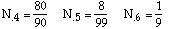In order to show that condition 2 holds, I must show that

PX <= P.4 = P.5 =P.6

where 0<= X <= 1 corresponds to some other strategy. This is easily done. For X > .6, PX = 0 by inspection. For .6 > X > .5, PX < P.6 by inspection. For .5 > X > .4, PX < P.5 by inspection. For .4 > X, PX < P.4 by inspection. So condition 2 is satisfied.

In order to consider condition 3, we need the common value of P.4, P.5, P.6. This is calculated as follows.

If two partners reach agreement, the average profit is .5. Otherwise it is 0. So the average profit is .5 x probability of agreement = .5 x (1 -Probability of no agreement). Agreement fails only if someone demanding .6 meets someone demanding .5 or .6.

So C = .5 (1 - N.6 x (N.6 + N.5 ) - N.5 x N.6)

= .5 (1 - N.6 (N.6 + 2N.5 ))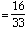To show that condition 3 is violated, I solve the equations for the more general case where all players demand either X, 1 - X, or .5; (X<= .5). This yieldsThe previous result corresponds to X = .4.

The average return, in this more general case, is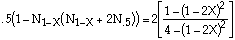which increases as X increases from 0 to .5 .

So the tentative solution (X = .4) can be continuously deformed (by increasing X) in a way that monotonically increases the average return. It so violates condition 3 and is not a solution.

I have considered only the case where N.4, N.5, N.6 > 0.

Additional distributions satisfying conditions 1 and 2 are:

N.4 = .8, N.5 = 0, N.6 = .2

which can be shown to violate condition 3 and:

N.4 = 0, N.5 = 1, N.6 = 0

In this last case the expected return is .5, its maximum possible value. There are no distributions with higher returns, hence this distribution cannot be deformed in any way that increases its return. Hence it satisfies condition 3 and is a solution. I now proceed to prove that it is the only solution.

THE GENERAL CASE

Definition: F(x) is a cumulative distribution <=>

1. F(x) is a non-decreasing function of x on (0, 1)

2.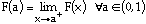3. F(0)=0

4. F(1)=1

comment: F(x) is the fraction of players demanding no more than x.

Definition: a cumulative distribution F(x) is a semi-solution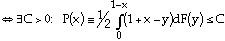F(x) is strictly increasing from below at a. (Henceforth I will write "strictly increasing" for "strictly increasing from below."

Comment: P(x) is the expected return per play to a player who demands x, when the distribution of players over strategies is F.

I now prove a series of lemmas in order to characterize the general semi-solution. Throughout the following F(x) is assumed to be a semi-solution and.

Definition: B is the set of points on (0,1) where F(x) is discontinuous or non-differentiable or where dF/dx is discontinuous.

Lemma 1: Points of B cannot be dense on any interval.

Proof: at a point of discontinuity F(x) must be strictly increasing. If F(x) is not differentiable, or dF/dx is not continuous, at a point, then F(x) is strictly increasing either at that point or on a set of points of which it is the limit. Were this not the case there would be a neighborhood of the point within which F(x) was constant.

It follows that if points of B are dense on the interval (a,b), then so are points where F(x) is strictly increasing. At any such point P(x) = C. Since F(x) is continuous from above,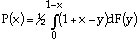is continuous from below. Hence.

Let x, x + [[Delta]] be two points in (a, b).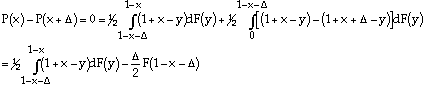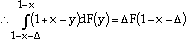Since this goes to 0 as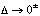, and since (l+x-y) > 0, it follows that F(y) must be continuous at l-x; since this holds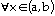it follows that F(x) is continuous on (l-b, 1-a)

Integrating by parts we find: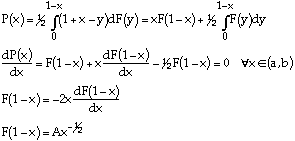Since F(x) is strictly increasing on (l-b, 1-a) we may repeat the same argument to show that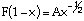on (a,b).

It follows that F(x) is continuous, differentiable, with a continuous derivative, on (a,b), contrary to the hypothesis.

Lemma 2: F(x) is strictly increasing at xo <=>F(x) is strictly increasing at l-xo,

Proof: Assume F(x) strictly increasing at xo but not at I-xo.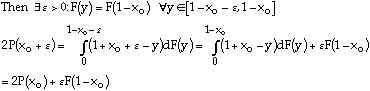f~0: F (y) =which is impossible.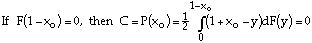which is impossible.

Hence F(x) is strictly increasing at l-xo. The converse is proved by applying this result, substituting l-xo for xo .

Lemma 3: If (a, b) contains no points of B, then either F(x) is constant on (a,b), (l-b,l-a), or else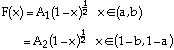Proof: at any point in (a,b), either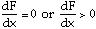By continuity, any interval within (a,b) in which points of zero derivative are dense contains in its interior only such points. By continuity of P(x), any interval within which points of positive derivative are dense contains in its interior only points for which P(x)= C. So (a,b) may be partitioned into open intervals in which dF/dx = 0, and open intervals in which P(x) = C. In any one of the latter, by the argument of lemma 1,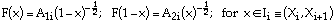But such a segment on (a,b) cannot be adjacent to another such segment with different A1i, by continuity of F, nor to a segment of constant F, by continuity of dF/dx, therefore either F(x) is constant on (a,b), or. In the former case F(x) must be constant on (1-b,1-a) by lemma 2; in the latter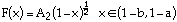by the argument of lemma 1. Q.E.D.

Lemma 4: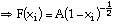Proof: consider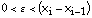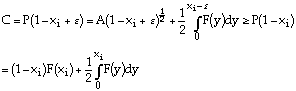Since F(x) is nondecreasing, we have: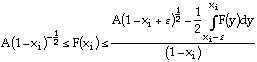for all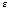however small.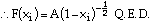Lemma 5:If F(x) is strictly increasing at xi the result follows immediately from lemma 2. Consider instead the case mentioned in lemma 1, where xi is the limit of points where F(x) is strictly increasing. It must be the limit from the right, else F(x) would be strictly increasing at xi. Hence by Lemma 2, l-xi is the limit from the left of points where F(x) is strictly increasing. Hence F(x) is strictly increasing at I-xi,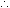at xi,P(xi) = P(1-xi) =C. Q.E.D.

Lemma 6: If xo is the greatest lower bound of points in B, then F(x) is discontinuous at xo and P(1-xo) = C.

Proof: By lemma 5 and left continuity of P(x), P(I-xo)=C.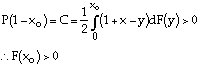It follows that either xo is a point of discontinuity or there is a point below it belonging to B (using lemma 3). The latter contradicts the assumption that xo is the glb of points in B.F(x) is discontinuous at xo. Q.E.D.

Lemma 7: F is discontinuous at only a countable number of points.

Proof: Since F is nondecreasing and goes from 0 to 1, it follows that there is at most one discontinuity of greater than 1/2, at most 2 of greater than a third and less than or equal to a half, at most three of greater than a fourth and less than or equal to a third, etc. Since the total set of discontinuities is the union of a countable collection of finite sets, it is itself countable. Q.E.D.

These seven lemmas permit me to characterize the general semi-solution and find its value C, as follows. Consider a semi-solution F. By lemmas 1, 6, and 7, (0,1) is partitioned by B into a countable number of intervals on whose interior F is continuous. By lemma 3,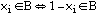.

Within each interval, by lemmas 2 and 3, either F(x) = constant, or F(x) = Ai (1-xi)-1/2; and F(x)=constant on (xi,x1+i)<=>F(x)=constant on (l-xi+1,I-xi). By lemma 4, P(xi)= P(1-xi) = C. Consider an interval (xi,xi+1) on which F(x) = constant.Consider an interval (xi,xi+1) on which F(x)=A(1-x)-1/2

By continuity from above (for F(x)) and lemma 4, F(x) = A(1-x)-1/2 on [xi,xi+1]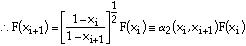I calculate C as follows:

Let xo be the least point in B (which exists by Lemma 6)ji=1 if F(x)=constant in (xi,xi+1), otherwise ji=2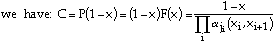It follows from the above that any semi-solution is uniquely characterized by its partition B together with a list L of the segments on which it is not constant. And it may be seen by inspection that any B and L generate a semi-solution, provided only that if xi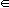B, so is 1 - xi, and that no two intervals in L be adjacent (by Lemma 4).

Definition: A solution is a semi-solution F(x) for which there exists no set of semi-solutions Fi(x) such that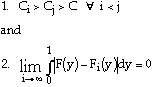Comment:is the average of the amount by which each player must alter his strategy in order to convert a distribution F(y) into a distribution Fi(y).

Theorem: The unique solution is

F(x)=0 0<= x<1/2

F(x)=1 1/2<x<= 1

Proof: Consider { xi} =B for sone semi-solution F(x). Create a set of new semi-solutions F(x,t), defining B(t)={ Xi(t)=(1-t)/2+txi} and writing L(t) such that (xi, xi+1)L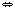(xi(t), xi+1(t))L(t). The value of F(x,t) we call C(t). I assume throughout that 0<= t<= 1; this guarantees that xi(t)(0,1) and that { xi(t)} has the same order as { xi}

I first show that C(t) increases as t decreases.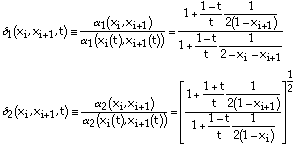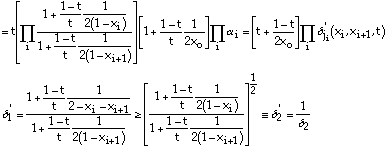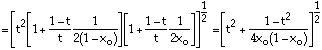since xo (l-xo) <1/4 for xo<1/2, it follows that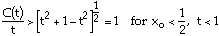By applying the same argument to G(x)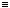F(x,tl) we see that, for xo < 1/2, C(t) increases monotonically as t decreases.

To prove that F(x) is not a solution if xo < 1/2, I require a { ti} : F(x,ti)Fi(x) satisfies the conditions for the Fi in the definition of a solution. If xo<1/2 the first condition is satisfied by choosing ti<tj<=>i<j. To satisfy the second condition, I will prove that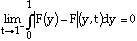It then suffices to choose any { ti} :I proceed as follows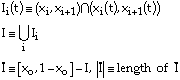It is easily shown that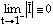Sincewhere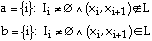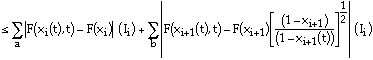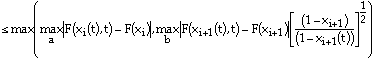since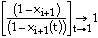I need only show that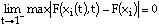in order to show that the integral over I goes to 0 as t->1-. To do so I use the explicit expression for a semi-solution previously derived.

F(1 - xo) =1 = F (1 - xo(t), t)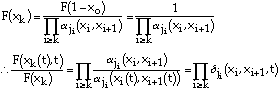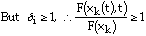and increases with decreasing k.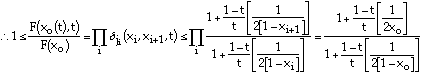which ->1+ as t->1-.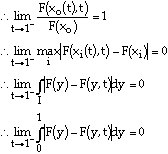if xo<1/2, F(x) is not a solutionThe unique solution is

F(x)=0 0<= x<= 1/2

F(x)=1 1/2<= x<= 1

Q.E.D.

Back to the list of articles.